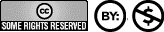### The LSD Tuning

##### The infrared spectrum of the LSD-25 molecule and its transcription into the auditory region.

The sound of molecules
Molecularly tuned music, like planetary tuned music, is composed according to strictly harmonic criteria, i.e. in harmony with given harmonic structures and on the basis of the natural laws of the "Cosmic Octave". Basis of the compositional elements of this music (tempo, rhythm, tone steps, intervals) are the physically measurable resonances of the molecules in the ultraviolet respectively in the infrared range (UV and IR range). The physically determined measured values are then octavated into the rhythmic range and into the audible tone range, thus forming the basis of the entire musical composition.

Electron spectroscopy - wavenumbers and wavelengths
By means of modern apparatus, electron spectroscopy can nowadays be used to perform the spectral analysis of chemical substances very precisely. Based on the measured wavenumbers (number of waves within a defined distance) or the measured wavelengths in the ultraviolet or infrared range, the spectra of different molecules can be precisely distinguished from each other. The wavenumbers and the corresponding wavelengths are inversely proportional to each other (function 1 / x).

The wavenumbers of molecules are usually given per centimeter (wavenumber / cm) in the literature. Wavelengths are generally listed in nanometers (1 nanometer = 1 millionth of a millimeter = 1 ten-millionth of a centimeter). To calculate the corresponding wavelength in nanometers (nm) from a wavenumber given per centimeter, one must first form the reciprocal (function 1 / x) of the wavenumber and then multiply the result by 10,000,000. The product obtained (result of the multiplication) is the measure of the wavelength in nanometers.

The infrared spectrum of the LSD-25 molecule
For example, the wavenumbers (number of waves per centimeter) of the resonances of the molecule lysergic acid diethylamide (LSD) in the IR spectrum are 750, 776, 850, 937, and 996. If we now form the reciprocal (1/x) of each of these numbers and then multiply each of these reciprocals by 10,000,000, we obtain the wavelengths ( λ ) in nanometers of the IR spectrum of the LSD molecule, as shown in the following table. Translated with www.DeepL.com/Translator (free version)

750 cm-1 . 1/x = 0.001 333 cm; λ 13 333 nm
776 cm-1 . 1/x = 0.001 289 cm; λ 12 887 nm
850 cm-1 . 1/x = 0.001 176 cm; λ 11 765 nm
937 cm-1 . 1/x = 0.001 067 cm; λ 10 672 nm
996 cm-1 . 1/x = 0.001 004 cm; λ 10 040 nm

The frequencies of the IR spectrum
Wavelengths and frequencies behave, as already mentioned elsewhere, in the electromagnetic range inversely proportional to each other, like also the time and the frequency, only with the difference that here still the speed of light is to be considered. It is valid:

λ = c / f and f = c / λ

where λ is the wavelength, f is the frequency, and c is the speed of light [2.997 925·1017nm·sec-1]

Thus, the frequencies of the maxima in the IR spectrum of the LSD molecule are:
2.998·1017nm·sec-1 / 13.333 nm = 2.248·1013 Hz
2.998·1017nm·sec-1 / 12.887 nm = 2.326·1013 Hz
2.998·1017nm·sec-1 / 11.765 nm = 2.548·1013 Hz
2.998·1017nm·sec-1 / 10.672 nm = 2.809·1013 Hz
2.998·1017nm·sec-1 / 10.040 nm = 2.986·1013 Hz

Octave into the audible range
The 37th sub-octaves of these five main frequencies of the LSD molecule are musically in the audible range of the middle (small) octave:

2.248·1013Hz / 237 = 163.6 Hz = E     violet
2.326·1013Hz / 237 = 169.3 Hz = E/F   red-violet
2.548·1013Hz / 237 = 185.4 Hz = F#    red
2.809·1013Hz / 237 = 204.4 Hz = G#  orange
2.986·1013Hz / 237 = 217.3 Hz = A     yellow-orange (yellow-orange)

Tuning data sheets
with further sub-octaves in the audible range, octaves in the visible range, as well as detailed tuning data (frequency numbers, cent values, microtunes, pitchwheel datas, microsteps, tempos, echo, reverb and loop times):

Data sheets for LSD tuning here as PDF2002, 2006 Hans Cousto Creative-Commons-Lizenz: